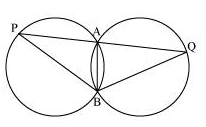Guru

# Two congruent circles intersect each other at points A and B. Through A any line segment PAQ is drawn so that P, Q lie on the two circles. Prove that BP = BQ. Q.9

• 0

What is the best way for solving the question from class 9th ncert math of Circles chapter of Ncert of exercise 10.6 of math. What is the best way for solving this question please guide me the best way for solving this question no.9 Two congruent circles intersect each other at points A and B. Through A any line segment PAQ is drawn so that P, Q lie on the two circles. Prove that BP = BQ.

Share

1. The diagram will beHere, ∠APB = ∠AQB (as AB is the common chord in both the congruent circles.)

Now, consider ΔBPQ,

∠APB = ∠AQB

So, the angles opposite to equal sides of a triangle.

∴ BQ = BP

• 0